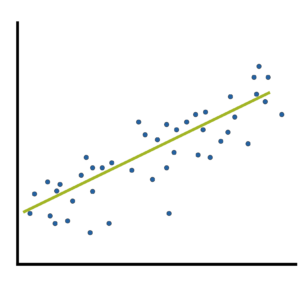# R-squared

### Two Types of Effect Size Statistic: Standardized and Unstandardized

June 26th, 2023 by

Effect size statistics are all the rage these days.

Journal editors are demanding them. Committees won’t pass dissertations without them.But the reason to compute them is not just that someone wants them — they can truly help you understand your data analysis.

### What Is an Effect Size Statistic?When many of us hear “Effect Size Statistic,” we immediately think we need one of a few statistics: Eta-squared, Cohen’s d, R-squared.
And yes, these definitely qualify. But the concept of an effect size statistic is actually much broader. Here’s a description from a nice article on effect size statistics:

“… information about the magnitude and direction of the difference between two groups or the relationship between two variables.

– Joseph A. Durlak, “How to Select, Calculate, and Interpret Effect Sizes”

If you think about it, many familiar statistics fit this description. Regression coefficients give information about the magnitude and direction of the relationship between two variables. So do correlation coefficients. (more…)

### The Difference Between R-squared and Adjusted R-squared

August 22nd, 2022 by

R², the the Coefficient of Determination, is one of the most useful and intuitive statistics we have in linear regression.It tells you how well the model predicts the outcome and has some nice properties. But it also has one big drawback.

### Measures of Model Fit for Linear Regression Models

May 9th, 2022 by

A well-fitting regression model results in predicted values close to the observed data values.The mean model, which uses the mean for every predicted value, generally would be used if there were no useful predictor variables. The fit of a proposed
regression model should therefore be better than the fit of the mean model. (more…)

### Simplifying a Categorical Predictor in Regression Models

January 14th, 2020 by

One of the many decisions you have to make when model building is which form each predictor variable should take. One specific version of thisdecision is whether to combine categories of a categorical predictor.

The greater the number of parameter estimates in a model the greater the number of observations that are needed to keep power constant. The parameter estimates in a linear (more…)

### Member Training: The Anatomy of an ANOVA Table

December 31st, 2019 byOur analysis of linear regression focuses on parameter estimates, z-scores, p-values and confidence levels. Rarely in regression do we see a discussion of the estimates and F statistics given in the ANOVA table above the coefficients and p-values.

And yet, they tell you a lot about your model and your data. Understanding the parts of the table and what they tell you is important for anyone running any regression or ANOVA model.

### Can a Regression Model with a Small R-squared Be Useful?

May 14th, 2012 byR² is such a lovely statistic, isn’t it?  Unlike so many of the others, it makes sense–the percentage of variance in Y accounted for by a model.

I mean, you can actually understand that.  So can your grandmother.  And the clinical audience you’re writing the report for.

A big is always good and a small one is always bad, right?

Well, maybe. (more…)